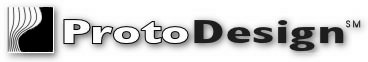###### Home | Products | Services | Support | Download | Order | Corporate | Site Map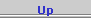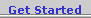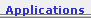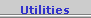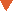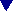## Application Programs for SansGUI

The following programs demonstrate scientific and engineering applications using the SansGUI Modeling and Simulation Environment.  Some of the programs are available for testing, and they may include source code for educational purposes.  Please check the descriptions of each program for details.

• Mixer - Solving a System of Linear Equations: demonstrates how to programmatically prepare necessary matrices and tables for a chemical reactor/mixer network with various concentrations and flow rates in the components, a typical engineering problem that involves a system of linear equations.  The equations are solved by using Naive Gauss Elimination or LU Decomposition algorithms, or calling the MATLAB engine from The Mathworks, Inc.

#### Mixer -- Solving a System of Linear Equations

• ##### Description

Project Mixer is a programming example that demonstrates how a simulator can be developed to solve a system of linear equations in a typical engineering application, using the SansGUI Modeling and Simulation Environment.  A mixer is a chemical reactor that takes a mixture from a number of input sources with various concentrations and flow rates and produces a homogeneous mixture with a new concentration.  It can be connected to other mixers, sources or sinks with in-flow and out-flow pipes.  Applying the law of mass conservation, this example shows how to set up and load a constant matrix and provide a table for the right hand side constants of the equations as well as a data column for the solutions.  A second  matrix is created to store the inverse matrix for further analyses.  The sizes of the matrices and the table are set through the program according to the number of mixers in a project model created by the user in the SansGUI Run-Time environment.

The user can select one of the three solution methods before a simulation run: 1) Naive Gauss Elimination, 2) LU Decomposition, or 3) Call MATLAB Engine.  The first two selections use the corresponding algorithms implemented in the simulator.  When 3) Call MATLAB Engine is chosen, the user needs to have the MATLAB software installed in his/her machine.  MATLAB can be obtained from The MathWorks, Inc.  Independent implementations of the project written in Microsoft Visual C/C++ and Compaq Visual Fortran are included with full source code.

• ##### Demonstration

Click on a picture to obtain its full size screen shot.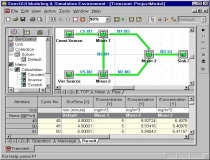This example model contains three mixers: Mixer-1 takes a constant source; Mixer-2 takes a variable source; Mixer-3 combines the output of the first two.  In this first example, the pipe from Mixer-2 to Mixer-1 is shut-off, with the flow rate set to 0.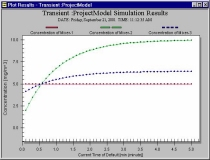With no in-flow from Mixer-2, Mixer-1 stays constant.  Both Mixer-2 and Mixer-3 have variable concentrations, affected by the variable source flows into Mixer-2..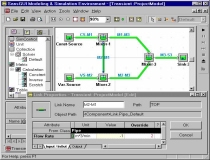In the second scenario, we "open" the pipe from Mixer-2 to Mixer-1 by setting the flow rate to 2 m3/min.  The output flow rates of the pipes from Mixer-1 and Mixer-2 to Mixer-3 are also modified to reflect the change.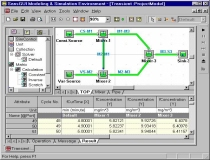Affected by the incoming flow from Mixer-2 and its variable source, Mixer-1 has its concentration changed through time in the second scenario.  The changing values are shown in the Result View in the Bottom Pane.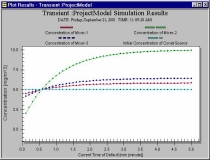This plot shows Mixer-1 has various concentrations through time after the pipe from Mixer-2 to Mixer-1 is opened.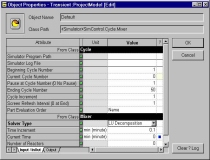The simulation user can select 1) Naive Gauss Elimination, 2) LU Decomposition, or 3) Calling MATLAB Engine in the simulation control object properties.  These options are created by the simulation developers using the SansGUI Development Environment.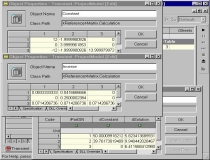A constant matrix, an inverse matrix, and a table that stores the right-hand-side (r-h-s) constants and the solution vector can be examined by the simulation user during and after a simulation run.  Because there are three mixers, the constant matrix is a 3x3 matrix.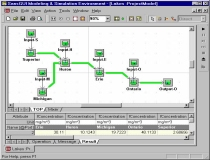This example shows a model of chloride concentrations in the Great Lakes, taken from an exercise problem in Chapra & Canale.  The solution is shown in the Result View in the Bottom Pane.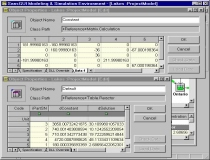The constant matrix and the table of r-h-s constants and solutions are examined.  These are created programmatically prior to calling the solution routines.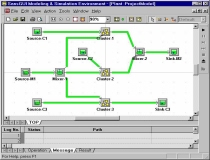This example shows a more elaborated model with three nearly identical subassemblies -- Cluster-1 through Cluster-3.  The first cluster was created manually while the second and the third clusters are replicas of the first one, created by using the Clone Part facility in SansGUI.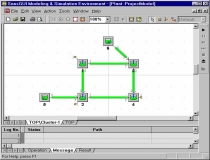Each cluster contains a subassembly depicted in this figure.  Three ports, two input ports and one output port, are exported from this subassembly for connections in the TOP assembly level.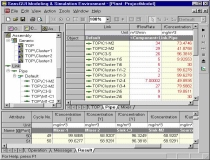The flow rates of all pipes (the data column with white background) can be entered in a Grid View in the Right Pane.  Also shown in the Grid View are the calculated concentrations of all pipes in the protected fields (light yellow background).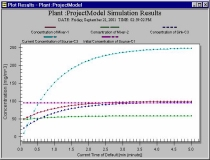The concentrations of some of the components through time are plotted.  These are selected from the data columns of the Result View in the previous figure.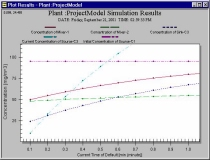The lower left corner of the previous plot is zoomed in to study the beginning minute of the simulation in more detail.  The user can select a rectangular area on the plot for multi-level zooming.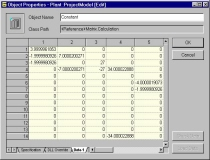The constant matrix of the example with multiple subassemblies is examined.  There are fourteen mixers in this model, yielding a 14x14 matrix.
• ##### Detail

A typical engineering problem involves a network of system components that can be modeled mathematically with a set of linear equations established with certain physical laws.  The unknowns in the equations represent the properties that the engineer would like to investigate, given a set of known conditions.  In this example, we use chemical reactors that mix conservative chemical fluid from input sources with various concentrations and dispense the new mixture to other mixers and/or sinks.  A pipe used to link two components controls the flow rate of the mixture that travels from one component to the other.  Normally, the sum of all the input flow rates is equal to the sum of all the output flow rates in a mixer so that the volume can stay constant.  The concentration in each mixer (assumed to be uniform) are the unknowns that we would like to solve.

With the SansGUI Modeling and Simulation Environment, the simulator is implemented in an object-oriented manner.  Using the SansGUI Run-Time Environment, instances of Mixers, Sources and Sinks can be created and connected with Pipes, derived from the Link class.  The linear equation solver is implemented in Class Solver, derived from Class Collection.  Three calculation matrices and a reactor table are reference objects used during the calculation process.  We implemented the Naive Gauss Elimination and LU Decomposition algorithms in C/C++ and Fortran independently.  Both C/C++ and Fortran implementation of the MATLAB engine calls are also provided to demonstrate how SansGUI can be used in conjunction with MATLAB.

• ##### Code

The implementation of this example can be found in the functions of seven classes: Base.Container.Reactor, Base.Container.Sink, Base.Source, Base.Source.Variable, Collection.Solver, Matrix.Calculation, and Table.Reactor.  Click on the following links to see all the necessary code for this project.  The C/C++ and Fortran implementations are independent; only one of them is required.

The entire project, including the program with its source code, on-line documentation, and the examples are available from our Download page.  This project requires the SansGUI Modeling and Simulation Environment.  A demonstration version of SansGUI (SGdemo) can be obtained from the same Download page.

• ##### Reference
• Chapra, Steven C. & Canale, Raymond P.  1998.  Numerical Methods for Engineers: with Programming and software Applications, 3rd Ed., WCB/McGraw-Hill, Boston, Massachusetts.

• The Mathworks, Inc.  1998.  MATLAB Application Programming Interface Guide, Version 5.0.  Natick, Massachusetts.  An External Interfaces/API Manual can be accessed on-line via The Mathworks' web site.

• ProtoDesign, Inc.  2001.  SansGUI Version 1.0 Document Set, Bolingbrook, Illinois.  The whole set of SansGUI Manuals can be accessed on-line.

• ##### Credit

This example is developed by the SansGUI Development Team at ProtoDesign, Inc.  The Great Lakes example is taken from Exercise 12.7 of Chapra & Canale.  The Fortran and C implementations of Naive Gauss Elimination and LU Decomposition use the algorithms introduced in Chapra & Canale's text.  MATLAB is a registered trademark of The Mathworks, Inc.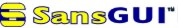Main Product Page